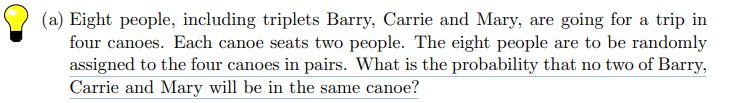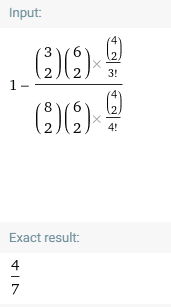# A simple probability question

Leo Liu
Homework Statement:
.
Relevant Equations:
.The answer is ##4/7##. I know the denominator which is number of unconstrained outcomes is $$\dfrac{\dbinom{8}{2}\dbinom{6}{2}\dbinom{4}{2}}{4!}$$But I am not sure how to find the number of outcomes under the constraint. Could some give me a hint? Thanks.

Mentor
2022 Award
Probabilities can often better be calculated if we consider the opposite event. How many possibilities are there, where two of three end up in the same canoe?

•Leo Liu
For the complement described by @fresh_42, it's just worth to note that you first have to choose 2 of Barry, Carrie and Mary, then you need to choose which of the 4 boats these two people go into, then you need to assign the remaining 6 people to the other 3 boats.

[You can work either by imagining the boats are distinguishable or indistinguishable, although I think it's conceptually easier to treat them as distinguishable.]

•Leo Liu
Homework Helper
Gold Member
2022 Award
Note that it doesn't make any difference in which order you select the people to be randomly assigned to canoes. So, another approach is to imagine that the triplets are assigned first.

Leo Liu
I got the following expression:
$$\xcancel{P(A)={1-\dfrac{\dbinom 3 2 \cdot 4\cdot \dfrac{\dbinom 6 2 \dbinom 4 2}{3!}}{\dfrac{\dbinom{8}{2}\dbinom{6}{2}\dbinom{4}{2}}{4!}}=\frac 4 7 }}$$
Thanks!

Last edited:
•PeroK
I got the following expression:
$$P(A)={1-\dfrac{\dbinom 3 2 \cdot 4\cdot \dfrac{\dbinom 6 2 \dbinom 4 2}{3!}}{\dfrac{\dbinom{8}{2}\dbinom{6}{2}\dbinom{4}{2}}{4!}}=\frac 4 7 }$$
Thanks!

I reckon it should be$$P(A) = 1 - \frac{ \dbinom 3 2 \cdot 4 \cdot \dbinom 6 2 \dbinom 4 2}{\dbinom 8 2 \dbinom 6 2 \dbinom 4 2} = \frac{4}{7}$$If you want, you could multiply by ##1 = (1/4!) / (1/4!)## to make it look like you're treating all the arrangements of the boats as indistinguishable.

•PeroK and Leo Liu
Homework Helper
Gold Member
2022 Award
It's a horrible way to do it in any case! If we put the triplets in first then the probability they all end up in different canoes is: $$p = 1 \times \frac 6 7 \times \frac 4 6 = \frac 4 7$$

•etotheipi and Leo Liu
Leo Liu
Yours is equal to ##-5/7##, I think.

See the note I added at the end of #6; it doesn't matter whether you take order into account for the boats. Doing so just amounts to dividing both the top and the bottom by ##4!##.
Sorry my bad. It appears bizarre to me that you get the probability even though you implicitly assign the order to boats by ##{\dbinom 8 2 \dbinom 6 2 \dbinom 4 2}##.

Leo Liu
I reckon it should be$$P(A) = 1 - \frac{ \dbinom 3 2 \cdot 4 \cdot \dbinom 6 2 \dbinom 4 2}{\dbinom 8 2 \dbinom 6 2 \dbinom 4 2} = \frac{4}{7}$$If you want, you could multiply by ##1 = (1/4!) / (1/4!)## to make it look like you're treating all the arrangements of the boats as indistinguishable.
Or maybe I shouldn't multiply the pair on the same boat by 4 since all boats are indistinguishable from one another?
$$P(A)={1-\dfrac{\dbinom 3 2 \cdot \cancel 4\cdot \dfrac{\dbinom 6 2 \dbinom 4 2}{3!}}{\dfrac{\dbinom{8}{2}\dbinom{6}{2}\dbinom{4}{2}}{4!}}=\frac 4 7}$$Sorry my bad. It appears bizarre to me that you get the probability even though you implicitly assign the order to boats by ##{\dbinom 8 2 \dbinom 6 2 \dbinom 4 2}##.

Say you have a bag with 1 red, 1 blue and 1 green chip in it. What's the probability you get 1 red and 1 blue, if you choose 2 chips in succession without replacement?

- If you treat it with order, you win with RB or BR - (2 ways), out of a total possible BR, RB, BG, GB, RG, GR - (6 ways).

- If you treat it without order, you win with {RB} - (1 way), out of a total possible {RB}, {BG}, {RG} - (3 ways).

Either way, the probability is 1/3. If the 'permutation factor ##N!##' is the same in the numerator and denominator, it doesn't matter whether you take into account ordering.

•Leo Liu
Staff Emeritus
Gold Member
It's a horrible way to do it in any case! If we put the triplets in first then the probability they all end up in different canoes is: $$p = 1 \times \frac 6 7 \times \frac 4 6 = \frac 4 7$$

I think you should really try to understand this solution.

Leo Liu
I think you should really try to understand this solution.
I don't actually get. Mind explaining? I followed the same idea and got ##4\cdot3\cdot2\quad 5\cdot4\cdot3\cdot\dbinom{2}{2}## as the numerator.

You put the first of your three special people in any seat, and then you have 7 seats left but only 6 into which you can put the second special person, due to the constraint of the special people needing to be in different boats. After seating the second special person, you have 6 seats left but only 4 into which you can put the third special person.

•Leo Liu
Leo Liu
You put the first of your 3 special people in any seat, and then you have 7 seats left but only 6 into which you can put the second of your 3 special people, due to the constraint of the special people needing to be in different boats. After seating the second of your 3 special people, you have 6 seats left but only 4 into which you can put the third of your 3 special people.
This is smart. Thanks for your explanation.
Meh, maybe I am too dumb to understand combinatorics and probability.

This is smart. Thanks for your explanation.
Meh, maybe I am too dumb to understand combinatorics and probability.

Don't thank me, it was @PeroK's idea!Homework Helper
Gold Member
2022 Award
This is smart. Thanks for your explanation.
Meh, maybe I am too dumb to understand combinatorics and probability.
Sometimes it's good to count, but sometimes it's better to go directly for the probabilities.

•Leo Liu